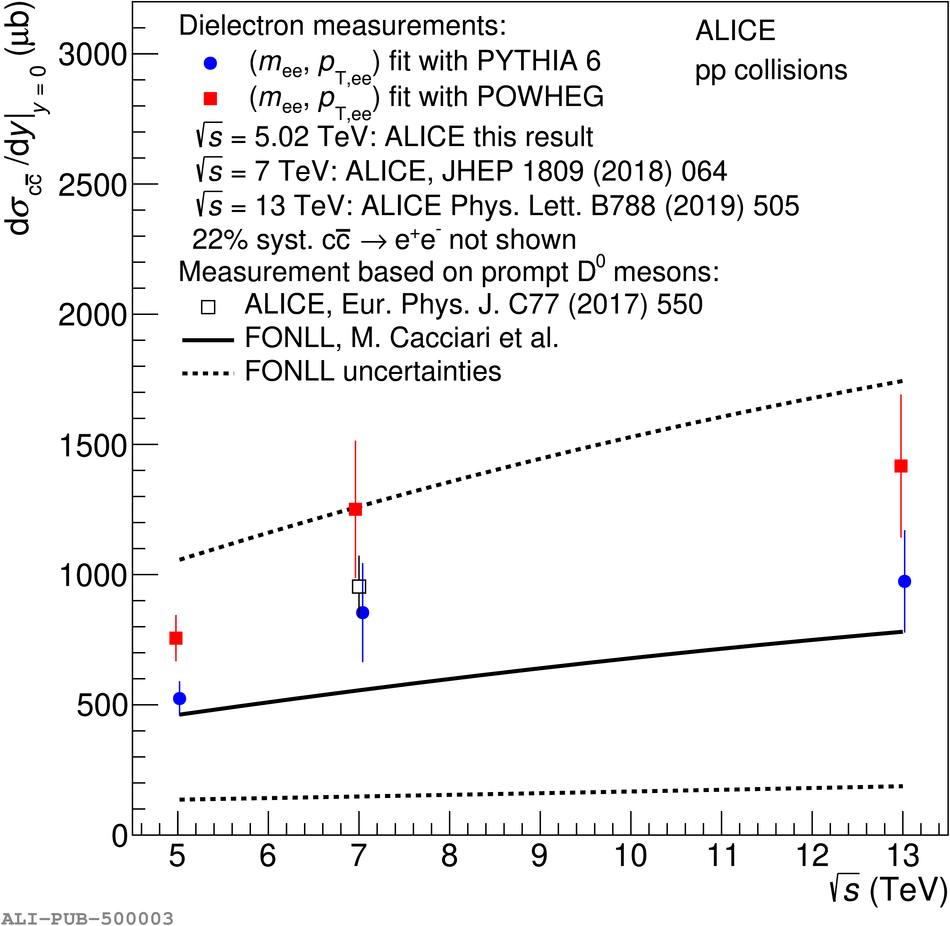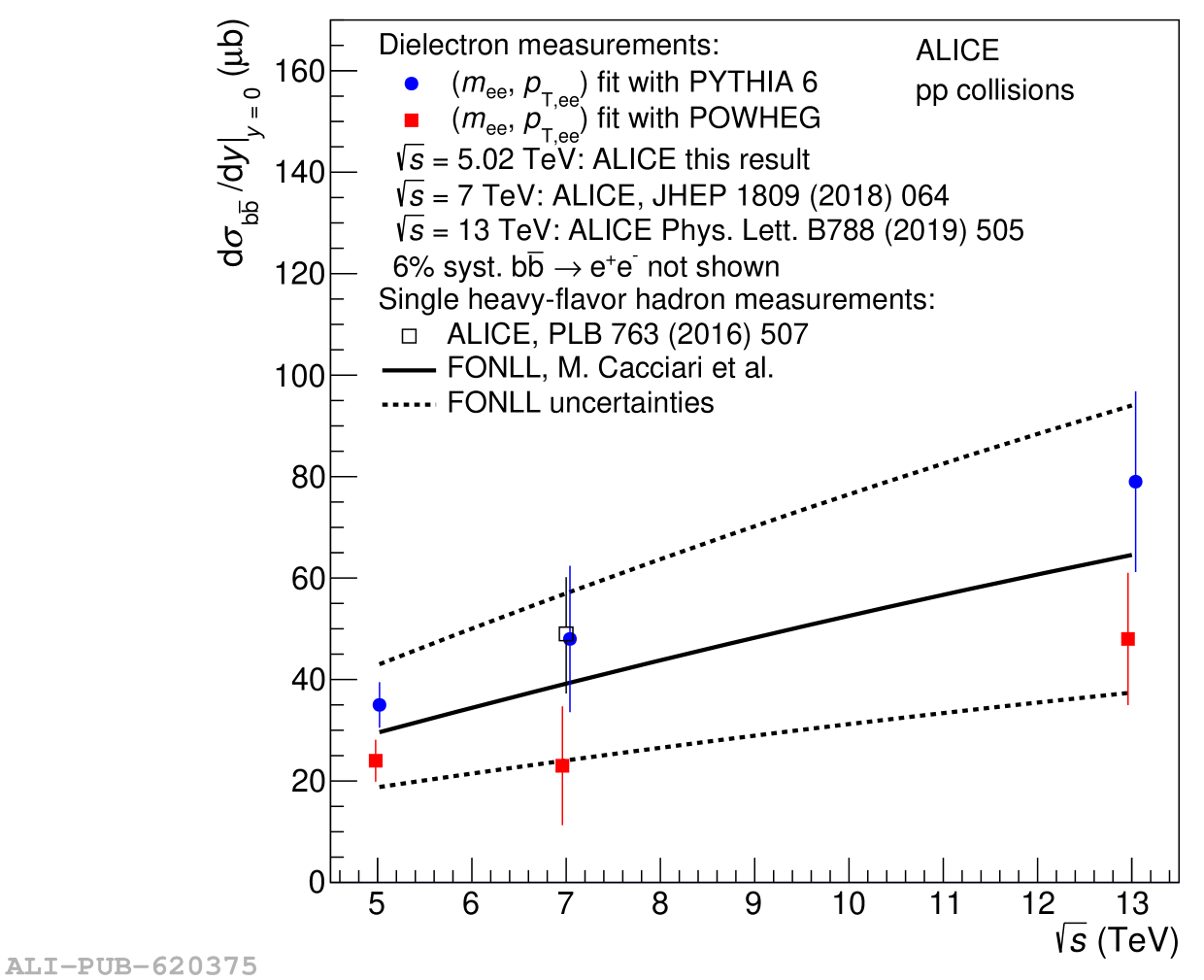# Figure 4

 Cross sections at midrapidity for c$\overline{\textrm{c}}$ (left) and b$\overline{\textrm{b}}$ (right) as a function of $\sqrt{s}$ in \pp collisions. The colored markers represent the measured midrapidity cross sections at \s = 5.02, 7, and 13 TeV which are derived using either PYTHIA 6 (blue circles) or POWHEG (red squares) simulations. The systematic and statistical uncertainty of the data points are summed in quadrature and represented by vertical bars. The measurements are compared with FONLL calculations (black solid line), with model uncertainties (dashed lines), and to single heavy-flavor hadron measurements (open markers). The referenced $\rm c\overline{c}$ cross section at \s = 7 TeV was obtained from a measurement of prompt $\rm D^0$ meson production with \ptMin{0} and \$|y|